# US7126759B2 - Zoom lens system - Google Patents

Zoom lens system Download PDF

## Info

Publication number
US7126759B2
US7126759B2 US10/892,169 US89216904A US7126759B2 US 7126759 B2 US7126759 B2 US 7126759B2 US 89216904 A US89216904 A US 89216904A US 7126759 B2 US7126759 B2 US 7126759B2
Authority
US
United States
Prior art keywords
lens
state
focal length
denotes
zoom
Prior art date
Legal status (The legal status is an assumption and is not a legal conclusion. Google has not performed a legal analysis and makes no representation as to the accuracy of the status listed.)
Active
Application number
US10/892,169
Other versions
US20050013015A1 (en
Inventor
Takayuki Sensui
Current Assignee (The listed assignees may be inaccurate. Google has not performed a legal analysis and makes no representation or warranty as to the accuracy of the list.)
Nikon Corp
Original Assignee
Nikon Corp
Priority date (The priority date is an assumption and is not a legal conclusion. Google has not performed a legal analysis and makes no representation as to the accuracy of the date listed.)
Filing date
Publication date
Priority to JP2003198644 priority Critical
Priority to JP2003-198644 priority
Priority to JP2004-198349 priority
Priority to JP2004198349A priority patent/JP4720117B2/en
Application filed by Nikon Corp filed Critical Nikon Corp
Assigned to NIKON CORPORATION reassignment NIKON CORPORATION ASSIGNMENT OF ASSIGNORS INTEREST (SEE DOCUMENT FOR DETAILS). Assignors: SENSUI, TAKAYUKI
Publication of US20050013015A1 publication Critical patent/US20050013015A1/en
Application granted granted Critical
Publication of US7126759B2 publication Critical patent/US7126759B2/en
Active legal-status Critical Current
Anticipated expiration legal-status Critical

## Links

• 239000011521 glass Substances 0.000 claims abstract description 66
• 239000000463 material Substances 0.000 claims abstract description 43
• 230000003287 optical Effects 0.000 claims abstract description 42
• 230000014509 gene expression Effects 0.000 claims description 58
• 230000004075 alteration Effects 0.000 description 48
• 238000010276 construction Methods 0.000 description 27
• 238000010586 diagram Methods 0.000 description 22
• 206010010071 Coma Diseases 0.000 description 8
• 201000009310 astigmatism Diseases 0.000 description 2
• 230000004048 modification Effects 0.000 description 2
• 238000006011 modification reaction Methods 0.000 description 2
• 238000003384 imaging method Methods 0.000 description 1
• 238000000926 separation method Methods 0.000 description 1
• 239000007787 solid Substances 0.000 description 1

## Images

•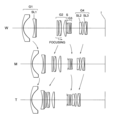•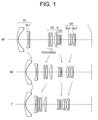•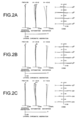•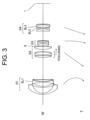•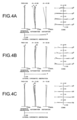••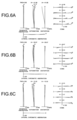•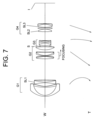•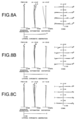••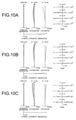•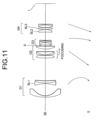•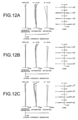•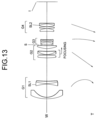•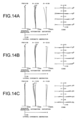•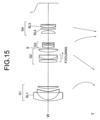•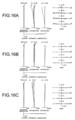•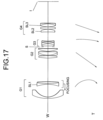•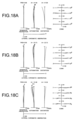•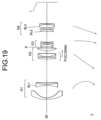•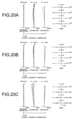••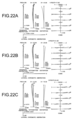## Classifications

• GPHYSICS
• G02OPTICS
• G02BOPTICAL ELEMENTS, SYSTEMS, OR APPARATUS
• G02B15/00Optical objectives with means for varying the magnification
• G02B15/14Optical objectives with means for varying the magnification by axial movement of one or more lenses or groups of lenses relative to the image plane for continuously varying the equivalent focal length of the objective
• G02B15/16Optical objectives with means for varying the magnification by axial movement of one or more lenses or groups of lenses relative to the image plane for continuously varying the equivalent focal length of the objective with interdependent non-linearly related movements between one lens or lens group, and another lens or lens group
• G02B15/177Optical objectives with means for varying the magnification by axial movement of one or more lenses or groups of lenses relative to the image plane for continuously varying the equivalent focal length of the objective with interdependent non-linearly related movements between one lens or lens group, and another lens or lens group having a negative front lens or group of lenses
• GPHYSICS
• G02OPTICS
• G02BOPTICAL ELEMENTS, SYSTEMS, OR APPARATUS
• G02B15/00Optical objectives with means for varying the magnification
• G02B15/14Optical objectives with means for varying the magnification by axial movement of one or more lenses or groups of lenses relative to the image plane for continuously varying the equivalent focal length of the objective
• G02B15/144Optical objectives with means for varying the magnification by axial movement of one or more lenses or groups of lenses relative to the image plane for continuously varying the equivalent focal length of the objective having four groups only
• G02B15/1445Optical objectives with means for varying the magnification by axial movement of one or more lenses or groups of lenses relative to the image plane for continuously varying the equivalent focal length of the objective having four groups only the first group being negative
• G02B15/144511Optical objectives with means for varying the magnification by axial movement of one or more lenses or groups of lenses relative to the image plane for continuously varying the equivalent focal length of the objective having four groups only the first group being negative arranged -+-+

## Abstract

A super-wide zoom lens system having sufficient zoom ratio and a high speed, capable of obtaining high optical performance. The system includes at least, in order from an object, a first lens group G1 having negative refractive power, and a second lens group G2 having positive refractive power. When zooming from a wide-angle end state to a telephoto end state, a distance between the first lens group G1 and the second lens group G2 varies. The first lens group G1 includes at least one negative lens element and a glass material constructing at least one negative lens element (SL1) in the first group G1 satisfies given conditions.

## Description

The disclosures of the following priority applications are herein incorporated by reference:
Japanese Patent Application No. 2003-198644 filed on Jul. 17, 2003 and
Japanese Patent Application No. 2004-198349 filed on Jul. 5, 2004.
BACKGROUND OF THE INVENTION
1. Field of the Invention
The present invention relates to a zoom lens system and in particular to a zoom lens system suitable for a single-lens-reflex camera having an angle of view of 75 degrees or more in a wide-angle end state and a zoom ratio of 3 or more.
2. Related Background Art
A zoom lens system having a negative lens group locating at the most object side (a so-called negative-lead type zoom lens) is easy to be made a wide-angle lens and has been made various proposals. On the other hand, the negative-lead type zoom lens has a drawback that it is difficult to satisfy both of a high zoom ratio and a high speed. Accordingly, high-speed zoom lenses overcoming the drawback have been proposed such as Japanese Patent Application Laid-Open Nos. 2000-241704 and 2000-221399.
However, the zoom lenses proposed by the patent documents do not satisfy both of a wide angle of view in the wide-angle end state and a high zoom ratio.
SUMMARY OF THE INVENTION
The present invention is made in view of the aforementioned problems and has an object to provide a super wide zoom lens system having a sufficient zoom ratio with a high speed capable of obtaining high optical performance.
According to one aspect of the present invention, a zoom lens system includes, in order from an object, a first lens group having negative refractive power, and a second lens group having positive refractive power. When the state of lens group positions varies from a wide-angle end state to a telephoto end state, each lens group is moved such that a distance between the first lens group and the second lens group varies. The first lens group includes at least one negative lens element. A glass material constructing at least one of the negative lens element among the at least one negative lens element in the first lens group satisfies all of the following three conditional expressions (1), (2) and (3):
67.0<ν  (1)
1.40<N  (2)
0<N+0.0032×ν−1.734  (3)
where ν denotes Abbe number of the glass material at d-line (λ=587.6 nm), and N denotes refractive index of the glass material at d-line (λ=587.6 nm).
In one preferred embodiment of the present invention, the zoom lens system further includes, in order from the object, a third lens group having negative refractive power locating to the image side of the second lens group, and a fourth lens group having positive refractive power. When the state of lens group positions varies from a wide-angle end state to a telephoto end state, each lens group is moved such that the distance between the first lens group and the second lens group decreases, a distance between the second lens group and the third lens group increases, and a distance between the third lens group and the fourth lens group decreases. The fourth lens group includes at least one positive lens element, and a glass material constructing at least one positive lens element among the at least one positive lens element in the fourth lens group satisfies all of the following three conditional expressions (1), (2), and (3):
67.0<ν  (1)
1.40<N  (2)
0<N+0.0032×ν−1.734  (3)
where ν denotes Abbe number of the glass material at d-line (λ=587.6 nm), and N denotes refractive index of the glass material at d-line (λ=587.6 nm).
In one preferred embodiment of the present invention, the following conditional expression (4) is preferably satisfied:
1.5<(−f1)/fw<2.3  (4)
where f1 denotes the focal length of the first lens group in a wide-angle end state, and fw denotes the focal length of the zoom lens system in a wide-angle end state.
In one preferred embodiment of the present invention, the following conditional expression (5) is preferably satisfied:
0.75<f2/(fw×ft)1/2<1.6  (5)
where f2 denotes the focal length of the second lens group, fw denotes the focal length of the zoom lens system in a wide-angle end state, and ft denotes the focal length of the zoom lens system in a telephoto end state.
In one preferred embodiment of the present invention, the following conditional expressions (6) and (7) are preferably satisfied:
0.6<(−f3)/f2<1.2  (6)
0.8<f4/(fw×ft)1/2<2.0  (7)
where f3 denotes the focal length of the third lens group, and f4 denotes the focal length of the fourth lens group.
In one preferred embodiment of the present invention, at least one lens element having negative refractive power with a concave surface facing to an image in the first lens group has an aspherical surface having negative refractive power getting weaker in accordance with increase in a distance to the surface from the optical axis.
In one preferred embodiment of the present invention, the negative lens element in the first lens group satisfying all of the three conditional expressions has a double concave shape.
In one preferred embodiment of the present invention, the first lens group includes the negative lens element that is constructed by a glass material satisfying all of the three conditional expressions and one other lens element, and the negative lens element is cemented with the other lens element.
In one preferred embodiment of the present invention, when the state of lens group positions varies from a wide-angle end state to a telephoto end state, each distance along the optical axis between each lens element composing the first lens group is fixed.
In one preferred embodiment of the present invention, the first lens group is composed of, in order from the object, a first sub-lens group having negative refractive power and a second sub-lens group having negative refractive power, and when the state of lens group positions varies from a wide-angle end state to a telephoto end state, the distance between the first sub-lens group and the second sub-lens group varies.
Other feature and advantages according to the present invention will be readily understood from the detailed description of the preferred embodiments in conjunction with the accompanying drawings.
BRIEF DESCRIPTION OF THE DRAWINGS
FIG. 1 is a diagram showing lens construction of a zoom lens system according to Example 1 of the present invention in a wide-angle end state (W), an intermediate focal length state (M), and a telephoto end state (T) together with lens group movement upon zooming.
FIGS. 2A, 2B and 2C graphically show various aberrations of the zoom lens system according to Example 1 in a wide-angle end state, an intermediate focal length state, and a telephoto end state, respectively, when the system is focusing at infinity.
FIG. 3 is a diagram showing lens construction of a zoom lens system according to Example 2 of the present invention.
FIGS. 4A, 4B and 4C graphically show various aberrations of the zoom lens system according to Example 2 in a wide-angle end state, an intermediate focal length state, and a telephoto end state, respectively, when the system is focusing at infinity.
FIG. 5 is a diagram showing lens construction of a zoom lens system according to Example 3 of the present invention.
FIGS. 6A, 6B and 6C graphically show various aberrations of the zoom lens system according to Example 3 in a wide-angle end state, an intermediate focal length state, and a telephoto end state, respectively, when the system is focusing at infinity.
FIG. 7 is a diagram showing lens construction of a zoom lens system according to Example 4 of the present invention.
FIGS. 8A, 8B and 8C graphically show various aberrations of the zoom lens system according to Example 4 in a wide-angle end state, an intermediate focal length state, and a telephoto end state, respectively, when the system is focusing at infinity.
FIG. 9 is a diagram showing lens construction of a zoom lens system according to Example 5 of the present invention.
FIGS. 10A, 10B and 10C graphically show various aberrations of the zoom lens system according to Example 5 in a wide-angle end state, an intermediate focal length state, and a telephoto end state, respectively, when the system is focusing at infinity.
FIG. 11 is a diagram showing lens construction of a zoom lens system according to Example 6 of the present invention.
FIGS. 12A, 12B and 12C graphically show various aberrations of the zoom lens system according to Example 6 in a wide-angle end state, an intermediate focal length state, and a telephoto end state, respectively, when the system is focusing at infinity.
FIG. 13 is a diagram showing lens construction of a zoom lens system according to Example 7 of the present invention.
FIGS. 14A, 14B and 14C graphically show various aberrations of the zoom lens system according to Example 7 in a wide-angle end state, an intermediate focal length state, and a telephoto end state, respectively, when the system is focusing at infinity.
FIG. 15 is a diagram showing lens construction of a zoom lens system according to Example 8 of the present invention.
FIGS. 16A, 16B and 16C graphically show various aberrations of the zoom lens system according to Example 8 in a wide-angle end state, an intermediate focal length state, and a telephoto end state, respectively, when the system is focusing at infinity.
FIG. 17 is a diagram showing lens construction of a zoom lens system according to Example 9 of the present invention.
FIGS. 18A, 18B and 18C graphically show various aberrations of the zoom lens system according to Example 9 in a wide-angle end state, an intermediate focal length state, and a telephoto end state, respectively, when the system is focusing at infinity.
FIG. 19 is a diagram showing lens construction of a zoom lens system according to Example 10 of the present invention.
FIGS. 20A, 20B and 20C graphically show various aberrations of the zoom lens system according to Example 10 in a wide-angle end state, an intermediate focal length state, and a telephoto end state, respectively, when the system is focusing at infinity.
FIG. 21 is a diagram showing lens construction of a zoom lens system according to Example 11 of the present invention.
FIGS. 22A, 22B and 22C graphically show various aberrations of the zoom lens system according to Example 11 in a wide-angle end state, an intermediate focal length state, and a telephoto end state, respectively, when the system is focusing at infinity.
DESCRIPTION OF THE PREFERRED EMBODIMENTS
A zoom lens system according to the present invention includes, in order from an object, a first lens group having negative refractive power, a second lens group having positive refractive power, a third lens group having negative refractive power, and a fourth lens group having positive refractive power. When the state of lens group positions varies from a wide-angle end state to a telephoto end state, each lens group is moved such that a distance between the first lens group and the second lens group decreases, a distance between the second lens group and the third lens group increases, and a distance between the third lens group and the fourth lens group decreases.
A glass material constructing at least one of negative lens elements in the first lens group and that constructing at least one of positive lens elements in the fourth lens group satisfy all of the following conditional expressions (1), (2), and (3):
67.0<ν  (1)
1.40<N  (2)
0<N+0.0032×ν−1.734  (3)
where ν denotes Abbe number of the glass material at d-line (λ=587.6 nm), and N denotes refractive index of the glass material at d-line (λ=587.6 nm).
In a zoom lens system according to the present invention, by constructing at least one of negative lens elements in the first lens group with a glass material satisfying the above-described conditional expressions, since lower ray among the off-axis rays passes through the periphery of the aforementioned negative lens, chromatic coma of the lower ray can be corrected well. The lower ray is a ray lower than the principal ray and an upper ray is a ray higher than the principal ray when the image height is positive.
The above-described conditional expressions (1), (2) and (3) are for appropriately setting refractive index and Abbe number of the glass material constructing at least one of negative lens elements in the first lens group.
When the glass material constructing at least one of negative lens elements in the first lens group does not satisfy any one of the above-described conditional expressions, negative distortion and lateral chromatic aberration in the wide-angle end state become difficult to be corrected with good balance. Moreover, in the wide-angle end state, chromatic coma produces to the lower ray side.
In a zoom lens system according to the present invention, by constructing at least one of positive lens elements in the fourth lens group with a glass material satisfying the above-described conditional expressions, since upper ray among the off-axis rays passes on the periphery of the aforementioned positive lens, chromatic coma of the upper ray can be corrected well.
The above-described conditional expressions (1), (2), and (3) are for appropriately setting refractive index and Abbe number of the glass material constructing at least one of positive lens element in the fourth lens group.
When the glass material constructing at least one of positive lens elements in the fourth lens group does not satisfy any one of the above-described conditional expressions, on-axis chromatic aberration and various aberrations in the telephoto end state become difficult to be corrected with good balance. Moreover, in the wide-angle end state, chromatic coma produces to the upper ray side.
Moreover, the glass material constructing at least one of negative lens elements in the first lens group preferably satisfies all of the following conditional expressions (1a), (2), and (3a):
67.0<ν<104.0  (1a)
1.40<N  (2)
0<N+0.0122×ν−2.5188  (3a)
where ν denotes Abbe number of the glass material at d-line (λ=587.6 nm), and N denotes refractive index of the glass material at d-line (λ=587.6 nm).
Moreover, the glass material constructing at least one of positive lens elements in the fourth lens group preferably satisfies all of the following conditional expressions (1a), (2), and (3a):
67.0<ν<104.0  (1a)
1.40<N  (2)
0<N+0.0122×ν−2.5188  (3a)
where ν denotes Abbe number of the glass material at d-line (λ=587.6 nm), and N denotes refractive index of the glass material at d-line (λ=587.6 nm).
Furthermore, a zoom lens system according to the present invention preferably satisfies the following conditional expression (4):
1.5<(−f1)/fw<2.3  (4)
where f1 denotes the focal length of the first lens group in a wide-angle end state, and fw denotes the focal length of the zoom lens system in a wide-angle end state.
Furthermore, a zoom lens system according to the present invention preferably satisfies the following conditional expression (5):
0.75<f2/(fw×ft)1/2<1.6  (5)
where f2 denotes the focal length of the second lens group, fw denotes the focal length of the zoom lens system in a wide-angle end state, and ft denotes the focal length of the zoom lens system in a telephoto end state.
Conditional expression (4) is for setting an appropriate range of the focal length of the first lens group in the wide-angle end state.
When the ratio (−f1)/fw is equal to or exceeds the upper limit of conditional expression (4), the focal length of the first lens group in the wide-angle end state becomes so long that the diameter of the front lens (the diameter of the lens element locating at the most object side) becomes difficult to be miniaturized.
On the other hand, when the ratio is equal to or falls below the lower limit of conditional expression (4), the focal length of the first lens group in the wide-angle end state becomes so short that distortion, coma, and curvature of field in the wide-angle end state become difficult to be corrected. Accordingly, power arrangement of the zoom lens system according to the present invention becomes difficult to be a telephoto type in the telephoto end state, so that it becomes difficult to secure the f-number in the telephoto end state.
Conditional expression (5) is for suitably setting the focal length of the second lens group.
When the ratio f2/(fw×ft)1/2 is equal to or exceeds the upper limit of conditional expression (5), the focal length of the second lens group becomes so long that the moving amount of the second lens group upon zooming becomes excessively long, so that it is undesirable. Moreover, the diameter of the aperture stop in the telephoto end state becomes so large increasing in the diameter of the lens barrel, so that it is undesirable.
On the other hand, when the ratio is equal to or falls below the lower limit of conditional expression (5), the focal length of the second lens group becomes excessively short, so it becomes difficult to correct coma and in particular spherical aberration in the telephoto end state.
In a zoom lens system according to the present invention, the following conditional expressions (6) and (7) are preferably satisfied:
0.6<(−f3)/f2<1.2  (6)
0.8<f4/(fw×ft)1/2<2.0  (7)
where f3 denotes the focal length of the third lens group, and f4 denotes the focal length of the fourth lens group.
Conditional expression (6) defines an appropriate range of the ratio of the focal length of the second lens group to that of the third lens group and is for securing the back focal length and high optical performance in a zoom lens system according to the present invention.
When the ratio (−f3)/f2 is equal to or exceeds the upper limit of conditional expression (6), the focal length of the third lens group becomes too long relative to that of the second lens group, so that it becomes difficult to secure the back focal length in the wide-angle end state.
On the other hand, when the ratio is equal to or falls below the lower limit of conditional expression (6), the focal length of the third lens group becomes too short relative to that of the second lens group producing large amount of spherical aberration, coma, and distortion in the third lens group, so that it becomes difficult to correct such aberrations with good balance.
Conditional expression (7) is for suitably setting the focal length of the fourth lens group.
When the ratio f4/(fw×ft)1/2 is equal to or exceeds the upper limit of conditional expression (7), the focal length of the fourth lens group becomes too long, so that it becomes difficult to secure the back focal length in the wide-angle end state as well as to secure sufficient zoom ratio.
On the other hand, when the ratio is equal to or falls below the lower limit of conditional expression (7), the focal length of the fourth lens group becomes too short producing large amount of variation in various aberrations upon zooming, so that it becomes difficult to correct aberrations over entire zoom range.
In a zoom lens system according to the present invention, it is preferable that at least one lens element having negative refractive power with a concave surface facing to an image in the first lens group has an aspherical surface having negative refractive power getting weaker in accordance with increase in a distance to the surface from the optical axis.
In a zoom lens system according to the present invention, in order to secure sufficient back focal length as well as to prevent the diameter of the front lens from getting larger, although it is effective to make refractive power of the first lens group larger, it also produces large amount of negative distortion. Accordingly, in a zoom lens system according to the present invention, an aspherical surface is arranged to a concave lens surface facing to the image in the first lens group, so the curvature of the lens surface can be smaller than that of the reference sphere. Moreover, the introduction of the aspherical surface produces little amount of spherical aberration and distortion, so that burden of aberration correction to lower lens elements (lens elements locating to the image side of the aspherical surface) can be lightened, so it is desirable.
In a zoom lens system according to the present invention, it is preferable that the negative lens element in the first lens group satisfying all of the three conditional expressions (1), (2), and (3) has a double concave shape. Generally, the glass material is soft in the hardness and tends to be deformed, so that it is preferable that the double concave shape can reduce the deformation.
Moreover, in a zoom lens system according to the present invention, it is preferable that the negative lens element that is constructed by a glass material satisfying all of the three conditional expressions (1), (2), and (3) is cemented with one other lens element. Generally, the glass material is soft in the hardness and tends to be deformed, so that it is preferable that cementing the negative lens with one other lens element can reduce the deformation.
Moreover, in a zoom lens system according to the present invention, it is preferable that when the state of lens group positions varies from a wide-angle end state to a telephoto end state, each distance along the optical axis between each lens element composing the first lens group is fixed. In this construction, it is preferable that the construction can be simplified and variation in aberration upon zooming becomes small.
Furthermore, in a zoom lens system according to the present invention, it is preferable that the first lens group is composed of, in order from the object, a first sub-lens group having negative refractive power and a second sub-lens group having negative refractive power, and when the state of lens group positions varies from a wide-angle end state to a telephoto end state, the distance between the first sub-lens group and the second sub-lens group varies. In this construction, variation in aberration upon zooming is not affected much and is corrected easily, so that it is preferable.
Each example according to the present invention will be explained below with reference to accompanying drawings.
EXAMPLE 1
FIG. 1 is a diagram showing lens construction of a zoom lens system according to Example 1 of the present invention in a wide-angle end state (W), an intermediate focal length state (M), and a telephoto end state (T) together with lens group movement upon zooming.
As shown in FIG. 1, a zoom lens system according to Example 1 includes, in order from an object, a first lens group G1 having negative refractive power, a second lens group G2 having positive refractive power, a third lens group G3 having negative refractive power, and a fourth lens group G4 having positive refractive power. When the state of lens group positions varies from a wide-angle end state to a telephoto end state, each lens group is moved such that a distance between the first lens group G1 and the second lens group G2 decreases, a distance between the second lens group G2 and the third lens group G3 increases, and a distance between the third lens group G3 and the fourth lens group G4 decreases.
An aperture stop S is arranged between the second lens group G2 and the third lens group G3 and is constructed such that the aperture stop S is moved along the optical axis in a body with the third lens group G3 upon zooming.
The above-described feature is common to all Examples explained below, so that the duplicated explanation will be left out in the following Examples.
In a zoom lens system according to Example 1, the second negative lens SL1 from the object side of the first lens group G1 and the first positive lens SL2 and the third positive lens SL3 from the object side of the fourth lens group G4 are made of the glass material (hereinafter called a “special glass” in the specification) satisfying the above-described conditional expressions (1), (2) and (3). The negative lens SL1 made of the special glass has a double concave shape and is cemented with the third lens counted from the object side. When the state of lens group positions varies from a wide-angle end state to a telephoto end state, each distance along the optical axis between each lens element composing the first lens group is fixed. This construction is common to Example 2 through Example 10 explained below, so that the duplicated explanation will be left out in the following Examples.
In a zoom lens system according to Example 1, focusing from infinity to a close object is carried out by moving a first lens from the object side of the second lens group G2 and a cemented lens constructed by a second lens cemented with a third lens from the object side of the second lens group G2 in a body along the optical axis to the image side.
Various values of a zoom lens system according to Example 1 are shown below in Table 1. In [Specifications], f denotes the focal length, FNO denotes an f-number, 2A denotes an angle of view, and BF denotes the back focal length.
In [Lens Data], the left most column shows the surface number that is a lens surface counted in order from the object, r denotes the radius of curvature of a lens, and d denotes a distance along the optical axis between the lens surfaces. N denotes refractive index of a medium between the lens surfaces at d-line (λ=587.56 nm) and ν denotes Abbe number of the medium between the lens surfaces at d-line (λ=587.56 nm).
In a zoom lens system according to each Example of the present invention, an aspherical surface is expressed by the following expression;
x=cy 2/[1+(1−κc 2 y 2)1/2 ]+Cy 4 +Cy 6 +C8·y 8 +C10·y 10 +C12·y 12
where y denotes a height from the optical axis, x denotes a sag amount, c denotes a radius of curvature of a reference sphere (a paraxial radius of curvature), κ denotes a conical coefficient, C4, C6, C8, C10 and C12 denote 4th, 6th, 8th, 10th and 12th order aspherical coefficient, respectively.
An aspherical surface is expressed in [Lens Data] by “*” attached to the surface number and the paraxial radius of curvature is listed on the column of radius of curvature “r”. In [Aspherical Data], conical coefficient “κ” and each aspherical coefficient are shown. In [Aspherical Data], “E-n” denotes “10−n”. For example, “1.234E-05” denotes “1.234×10−5”.
In “Each Lens Block Magnification”, β denotes an imaging magnification between object and image. “1-POS” shows when the system is focusing at infinity in a wide-angle end state, “2-POS” shows when the system is focusing at infinity in an intermediate focal length state, “3-POS” shows when the system is focusing at infinity in a telephoto end state, “4-POS” shows when the system is focusing at β=−0.02500 in the wide-angle end state, “5-POS” shows when the system is focusing at β=−0.02500 in the intermediate focal length state, “6-POS” shows when the system is focusing at β=−0.02500 in the telephoto end state, “7-POS” shows when the system is focusing at a close object in the wide-angle end state, “8-POS” shows when the system is focusing at a close object in the intermediate focal length state, “9-POS” shows when the system is focusing at a close object in the telephoto end state. “B(m-n)” denotes a lens block formed by lens elements locating between the “m-th” lens surface and the “n-th” lens surface.
In the tables for various values, “mm” is generally used for the unit of length such as the focal length, the radius of curvature, and the separation between optical surfaces. However, since an optical system proportionally enlarged or reduced its dimension can be obtained similar optical performance, the unit is not necessary to be limited to “mm” and any other suitable unit can be used.
The explanation of reference symbols is the same in the other examples.
 TABLE 1 [Specifications]
 Wide-angle Intermediate Telephoto
 f = 16.5 30.67 49.5 2A = 86.8 51.1 33.1° FNO = 2.89
 [Lens Data]
 Surface Number r d N ν
 1) 76.2729 2.5000 1.744429 49.52 * 2) 23.2876 22.7082 3) −64.3264 1.3000 1.592400 68.33 SL1 4) 94.5578 2.9406 1.805180 25.43 5) 2047.8525 D5 * 6) 212.8974 3.1597 1.677900 55.34 7) −110.2891 0.1000 8) 122.3160 1.0000 1.846660 23.78 9) 34.9545 5.0514 1.618000 63.38 10) −171.3120 0.9881 *11) 50.7936 5.3000 1.739929 49.25 12) −290.3704 D12
 13> 1.2 Aperture Stop S
 14) 185.3843 3.7000 1.846660 23.78 15) −28.5773 1.0000 1.804000 46.58 16) 110.0860 1.9000 17) −41.1906 1.0000 1.804000 46.58 18) 84.5433 D18 19) −115.2418 5.0000 1.569070 71.31 SL2 20) −34.7029 0.1000 21) −1329.5201 4.0000 1.618000 63.38 22) −42.2832 0.1000 23) 48.4541 5.3788 1.497820 82.52 SL3 24) −32.1426 1.0000 1.846660 23.78 25) −732.5906
 [Aspherical Data]
 Surface Number 2 κ = 0.0000 C4 = 4.05250E−06 C6 = 6.11040E−10 C8 = 8.18800E−12 C10 = −1.46560E−14 C12 = 0.11470E−16 Surface Number 6 κ = 1.0000 C4 = −2.09280E−06 C6 = 1.24370E−09 C8 = 1.65820E−12 C10 = 0.00000E+00 Surface Number 11 κ = 1.0000 C4 = 1.82870E−06 C6 = −7.99320E−10 C8 = 5.20040E−13 C10 = −1.71450E−15
 [Variable Intervals]
 Wide-angle Intermediate Telephoto
 D5 43.77360 14.31030 2.37600 D12 1.35000 14.04720 25.98790 D18 18.19420 11.45430 3.46290
 [Each Lens Block Magnification]
 1-POS 2-POS 3-POS
 B(1–5) 0.00000 0.00000 0.00000 B(6–10) −4.23522 6.58245 3.23525 B(11–12) 0.13138 −0.15738 −0.49191 B(13–18) −4.41877 −2.81762 −1.70363 B(19–25) −0.21883 −0.34259 −0.59534
 4-POS 5-POS 6-POS
 β −0.02500 −0.02500 −0.02500 B(1–5) 0.04712 0.02509 0.01550 B(6–10) −4.21836 6.59060 3.24195 B(11–12) 0.13131 −0.15745 −0.49197 B(13–18) −4.41638 −2.81665 −1.70331 B(19–25) −0.21893 −0.34269 −0.59544
 7-POS 8-POS 9-POS
 β −0.062 −0.1100 −0.180 B(1–5) 0.11999 0.11168 0.11218 B(6–10) −4.20477 6.58519 3.27364 B(11–12) 0.13094 −0.15875 −0.49523 B(13–18) −4.40373 −2.79858 −1.68681 B(19–25) −0.21949 −0.34464 −0.60085
FIGS. 2A, 2B and 2C graphically show various aberrations of the zoom lens system according to Example 1 in a wide-angle end state, an intermediate focal length state, and a telephoto end state, respectively, when the system focusing at infinity.
In respective graphs, FNO denotes the f-number, and A denotes a half angle of view (unit: degree). In the graphs showing astigmatism and distortion, the maximum value of a half angle of view A is shown. Moreover, “d” and “g” denote aberrations at d-line (λ=587.6 nm) and g-line (λ=435.8 nm), respectively.
In the graph showing spherical aberration, FNO shows the value at the maximum aperture. In the graph showing astigmatism, a solid line indicates a sagittal image plane and a broken line indicates a meridional image plane.
In the graph showing coma, a half angle of view A is shown. The above-described explanation regarding various aberration graphs is the same as the other examples.
As is apparent from the respective graphs, the zoom lens system according to Example 1 shows superb optical performance as a result of good corrections to various aberrations in each focal length state (the wide-angle end state, the intermediate focal length state, and the telephoto end state).
EXAMPLE 2
FIG. 3 is a diagram showing lens construction of a zoom lens system according to Example 2 of the present invention. By the way, the figures showing lens construction of the following each Example shows only in the wide-angle end state (W).
In a zoom lens system according to Example 2, the third negative lens SL1 from the object side of the first lens group G1 and the second positive lens SL2 and the third positive lens SL3 from the object side of the fourth lens group G4 are made of the special glass. The negative lens SL1 made of the special glass has a double concave shape.
In a zoom lens system according to Example 2, focusing from infinity to a close object is carried out by moving a first lens from the object side of the second lens group G2 and a cemented lens constructed by a second lens cemented with a third lens from the object side of the second lens group G2 in a body along the optical axis to the image side.
Various values of a zoom lens system according to Example 2 are shown below in Table 2.
 TABLE 2 [Specifications]
 Wide-angle Intermediate Telephoto
 f = 17.55 30.81 53.4 2A = 83.38 51.18 30.96° FNO = 2.89
 [Lens Data]
 Surface Number r d N ν
 1) 43.0102 2.0000 1.620410 60.29 2) 21.3899 11.2854 3) 59.7338 2.0000 1.744429 49.55 * 4) 32.3245 8.1026 5) −65.6686 1.3000 1.569070 71.31 SL1 6) 96.9021 0.2000 7) 69.0297 3.3904 1.805180 25.43 8) 426.3566 D8 * 9) 92.4945 3.4356 1.677900 55.34 10) −186.1620 0.1000 11) −1876.6557 1.0000 1.805180 25.43 12) 38.6412 6.0000 1.640000 59.69 13) −79.2843 1.0018 *14) 49.2354 5.9545 1.637055 58.25 15) −123.3871 D15
 16> 1.2 Aperture Stop S
 17) 121.9585 3.6731 1.846660 23.78 18) −31.0894 1.0000 1.804000 46.58 19) 35.1118 3.2174 20) −26.8809 1.0000 1.804000 46.58 21) −65.7049 D21 22) −197.0467 4.6157 1.618000 63.38 23) −32.6618 0.1000 24) 173.9124 2.3568 1.497820 82.52 SL2 25) −118.9824 0.1000 26) 89.9876 5.0362 1.497820 82.52 SL3 27) −27.9105 1.0000 1.846660 23.78 28) −77.7117
 [Aspherical Data]
 Surface Number 4 κ = 0.0000 C4 = −2.80900E−06 C6 = −7.05870E−09 C8 = 1.85210E−11 C10 = −7.69200E−14 C12 = 0.54670E−16 Surface Number 9 κ = 1.0000 C4 = −3.21370E−06 C6 = 3.94540E−09 C8 = −7.52750E−13 C10 = 0.00000E+00 Surface Number 14 κ = 1.0000 C4 = 1.20020E−06 C6 = −2.72780E−09 C8 = 2.23320E−12 C10 = −3.44650E−15
 [Variable Intervals]
 Wide-angle Intermediate Telephoto
 D8 43.73070 16.11230 1.75000 D15 1.35000 12.50760 25.54550 D21 18.86450 14.53890 5.68120
 [Each Lens Block Magnification]
 1-POS 2-POS 3-POS
 B(1–8) 0.00000 0.00000 0.00000 B(9–13) −3.50130 9.83804 3.30002 B(14–15) 0.15216 −0.09599 −0.47835 B(16–21) −4.93806 −3.44613 −1.79102 B(22–28) −0.21648 −0.30726 −0.61284
 4-POS 5-POS 6-POS
 β −0.02500 −0.02500 −0.02500 B(1–8) 0.04455 0.02510 0.01444 B(9–13) −3.48253 9.84378 3.30667 B(14–15) 0.15210 −0.09605 −0.47840 B(16–21) −4.93546 −3.44484 −1.79070 B(22–28) −0.21658 −0.30736 −0.61294
 7-POS 8-POS 9-POS
 β −0.066 −0.111 −0.195 B(1–8) 0.12046 0.11279 0.11330 B(9–13) −3.46019 9.78411 3.34074 B(14–15) 0.15174 −0.09720 −0.48185 B(16–21) −4.91969 −3.42048 −1.77110 B(22–28) −0.21720 −0.30930 −0.61916
FIGS. 4A, 4B and 4C graphically show various aberrations of the zoom lens system according to Example 2 in a wide-angle end state, an intermediate focal length state, and a telephoto end state, respectively, when the system focusing at infinity.
As is apparent from the respective graphs, the zoom lens system according to Example 2 shows superb optical performance as a result of good corrections to various aberrations in each focal length state (the wide-angle end state, the intermediate focal length state, and the telephoto end state).
EXAMPLE 3
FIG. 5 is a diagram showing lens construction of a zoom lens system according to Example 3 of the present invention.
In a zoom lens system according to Example 3, the third negative lens SL1 from the object side of the first lens group G1 and the first positive lens SL2 and the third positive lens SL3 from the object side of the fourth lens group G4 are made of the special glass. The negative lens SL1 made of the special glass has a double concave shape and is cemented with the fourth lens counted from the object side.
In a zoom lens system according to Example 3, focusing from infinity to a close object is carried out by moving a cemented lens constructed by a first lens cemented with a second lens from the object side of the second lens group G2 and a cemented lens constructed by a third lens cemented with a fourth lens from the object side of the second lens group G2 in a body along the optical axis to the image side.
Various values of a zoom lens system according to Example 3 are shown below in Table 3.
 TABLE 3 [Specifications]
 Wide-angle Intermediate Telephoto
 f = 17.55 29.61 53.4 2A = 83.36 53.34 31.1° FNO = 2.89
 [Lens Data]
 Surface Number r d N ν
 1) 41.7490 2.0000 1.799520 42.24 2) 22.5226 14.4873 3) 74.2071 2.0000 1.796681 45.37 * 4) 38.7933 8.0658 5) −92.4063 1.3000 1.569070 71.31 SL1 6) 58.4359 3.7360 1.846660 23.78 7) 289.3259 D7 8) 180.6275 4.2583 1.696800 55.52 9) −50.6496 1.0000 1.846660 23.78 10) −80.4575 0.1000 11) 41.9083 1.0000 1.846660 23.78 12) 25.4611 6.0000 1.487490 70.24 13) 157.3587 1.0024 *14) 43.2622 5.7575 1.713000 53.85 15) −259.6633 D15
 16> 1.2 Aperture Stop S
 17) 128.9726 4.3615 1.846660 23.78 18) −24.3308 1.0000 1.804000 46.58 19) 49.4407 2.5995 20) −31.3713 1.0000 1.804000 46.58 21) 115.9859 D21 22) 368.8076 5.0000 1.497820 82.52 SL2 23) −28.1873 0.1000 24) 159.2657 2.7000 1.618000 63.38 25) −123.3241 0.1000 26) 65.0663 5.7301 1.497820 82.52 SL3 27) −28.1289 1.0000 1.846660 23.78 28) −106.6177
 [Aspherical Data]
 Surface Number 4 κ = 0.0000 C4 = −3.19380E−06 C6 = −4.94320E−09 C8 = 7.51060E−13 C10 = −1.32410E−14 C12 = −0.44693E−17 Surface Number 14 κ = 1.0000 C4 = 1.00000E−08 C6 = 3.75250E−10 C8 = 1.08920E−12 C10 = 0.00000E+00
 [Variable Intervals]
 Wide-angle Intermediate Telephoto
 D7 39.33590 16.23690 1.75000 D15 1.35000 10.85920 22.23130 D21 17.07340 12.51060 5.52610
 [Each Lens Block Magnification]
 1-POS 2-POS 3-POS
 B(1–7) 0.00000 0.00000 0.00000 B(8–13) −3.80389 12.59026 3.40003 B(14–15) 0.14366 −0.07072 −0.43257 B(16–21) −2.78220 −2.33000 −1.35946 B(22–28) −0.38982 −0.48203 −0.90196
 4-POS 5-POS 6-POS
 β −0.02500 −0.02500 −0.02500 B(1–7) 0.04277 0.02511 0.01387 B(8–13) −3.78623 12.59237 3.40648 B(14–15) 0.14360 −0.07077 −0.43262 B(16–21) −2.78119 −2.32934 −1.35927 B(22–28) −0.38994 −0.48215 −0.90208
 7-POS 8-POS 9-POS
 β −0.066 −0.107 −0.197 B(1–7) 0.11503 0.10862 0.10999 B(8–13) −3.76723 12.47409 3.43905 B(14–15) 0.14323 −0.07178 −0.43562 B(16–21) −2.77512 −2.31783 −1.34763 B(22–28) −0.39065 −0.48422 −0.90944
FIGS. 6A, 6B and 6C graphically show various aberrations of the zoom lens system according to Example 3 in a wide-angle end state, an intermediate focal length state, and a telephoto end state, respectively, when the system focusing at infinity.
As is apparent from the respective graphs, the zoom lens system according to Example 3 shows superb optical performance as a result of good corrections to various aberrations in each focal length state (the wide-angle end state, the intermediate focal length state, and the telephoto end state).
EXAMPLE 4
FIG. 7 is a diagram showing lens construction of a zoom lens system according to Example 4 of the present invention.
In a zoom lens system according to Example 4, the third negative lens SL1 from the object side of the first lens group G1 and the first positive lens SL2 and the third positive lens SL3 from the object side of the fourth lens group G4 are made of the special glass. The negative lens SL1 made of the special glass has a double concave shape and is cemented with the fourth lens counted from the object side.
In a zoom lens system according to Example 4, focusing from infinity to a close object is carried out by moving a cemented lens constructed by a first lens cemented with a second lens from the object side of the second lens group G2 and a cemented lens constructed by a third lens cemented with a fourth lens from the object side of the second lens group G2 in a body along the optical axis to the image side.
Various values of a zoom lens system according to Example 4 are shown below in Table 4.
 TABLE 4 [Specifications]
 Wide-angle Intermediate Telephoto
 f = 17.55 30.79 53.4 2A = 83.34 51.24 30.98° FNO = 2.89
 [Lens Data]
 Surface Number r d N ν
 1) 40.9179 2.0000 1.806100 40.94 2) 21.9529 14.9387 3) 85.5860 2.0000 1.796681 45.37 * 4) 41.8649 6.6331 5) −88.8627 1.3000 1.569070 71.31 SL1 6) 79.4414 3.4131 1.846660 23.78 7) 4482.0238 D7 8) 265.6240 4.1124 1.696800 55.52 9) −53.0226 1.0000 1.805180 25.43 10) −85.4782 0.1000 11) 48.4579 1.0000 1.846660 23.78 12) 28.3332 6.0000 1.487490 70.24 13) 609.7882 1.0047 14) 44.0030 5.8155 1.696800 55.52 15) −207.9680 D15
 16> 1.2 Aperture Stop S
 17) 132.9964 4.2981 1.846660 23.78 18) −24.6683 1.0000 1.804000 46.58 19) 52.5868 2.6051 20) −31.0252 1.0000 1.804000 46.58 21) 108.4429 D21 22) 1202.2229 5.0000 1.497820 82.52 SL2 23) −26.9875 0.1000 24) 152.4677 2.7000 1.618000 63.38 25) −129.6517 0.1000 26) 54.2036 6.0000 1.497820 82.52 SL3 27) −30.0273 1.0000 1.846660 23.78 28) −162.9006
 [Aspherical Data]
 Surface Number 4 κ = 0.0000 C4 = −4.05780E−06 C6 = −3.17270E−09 C8 = −1.99470E−11 C10 = 5.77110E−14 C12 = −0.94474E−16
 [Variable Intervals]
 Wide-angle Intermediate Telephoto
 D7 40.95880 15.53650 1.75000 D15 1.35000 12.25530 23.90170 D21 17.41550 13.09280 6.05810
 [Each Lens Block Magnification]
 1-POS 2-POS 3-POS
 B(1–7) 0.00000 0.00000 0.00000 B(8–13) −3.82942 10.11327 3.40001 B(14–15) 0.13758 −0.08740 −0.41103 B(16–21) −2.61985 −2.38465 −1.57100 B(22–28) −0.41289 −0.47443 −0.78981
 4-POS 5-POS 6-POS
 β −0.02500 −0.02500 −0.02500 B(1–7) 0.04449 0.02510 0.01443 B(8–13) −3.81121 10.11878 3.40667 B(14–15) 0.13752 −0.08746 −0.41108 B(16–21) −2.61895 −2.38397 −1.57075 B(22–28) −0.41301 −0.47455 −0.78993
 7-POS 8-POS 9-POS
 β −0.066 −0.111 −0.195 B(1–7) 0.12027 0.11285 0.11327 B(8–13) −3.79169 10.05288 3.44006 B(14–15) 0.13714 −0.08853 −0.41394 B(16–21) −2.61348 −2.37106 −1.55587 B(22–28) −0.41373 −0.47678 −0.79712
FIGS. 8A, 8B and 8C graphically show various aberrations of the zoom lens system according to Example 4 in a wide-angle end state, an intermediate focal length state, and a telephoto end state, respectively, when the system focusing at infinity.
As is apparent from the respective graphs, the zoom lens system according to Example 4 shows superb optical performance as a result of good corrections to various aberrations in each focal length state (the wide-angle end state, the intermediate focal length state, and the telephoto end state).
EXAMPLE 5
FIG. 9 is a diagram showing lens construction of a zoom lens system according to Example 5 of the present invention.
In a zoom lens system according to Example 5, the second negative lens SL1 from the object side of the first lens group G1 and the first positive lens SL2 and the third positive lens SL3 from the object side of the fourth lens group G4 are made of the special glass. The negative lens SL1 made of the special glass has a double concave shape and is cemented with the third lens counted from the object side.
In a zoom lens system according to Example 5, focusing from infinity to a close object is carried out by moving a first lens from the object side of the second lens group G2 and a cemented lens constructed by a second lens cemented with a third lens from the object side of the second lens group G2 in a body along the optical axis to the image side.
Various values of a zoom lens system according to Example 5 are shown below in Table 5.
 TABLE 5 [Specifications]
 Wide-angle Intermediate Telephoto
 f = 17.55 31.43 52.7 2A = 83.30 50.04 31.16° FNO = 2.89
 [Lens Data]
 Surface Number r d N ν
 1) 68.8329 2.5000 1.744429 49.55 * 2) 23.7109 22.4287 3) −58.4517 1.3000 1.518601 69.98 SL1 4) 70.0469 2.9936 1.805180 25.43 5) 243.9094 D5 * 6) 140.0441 3.4072 1.677900 55.34 7) −118.9794 0.1000 8) 124.6930 1.0000 1.846660 23.78 9) 34.2030 5.1696 1.618000 63.38 10) −189.4107 4.8624 *11) 53.9597 5.3000 1.739929 49.25 12) −211.8800 D12
 13> 1.2 Aperture Stop S
 14) 172.5088 4.0000 1.846660 23.78 15) −28.2347 1.0000 1.804000 46.58 16) 89.6343 1.9000 17) −39.5906 1.0000 1.804000 46.58 18) 99.5109 D18 19) −134.6632 2.9801 1.497820 82.52 SL2 20) −28.2015 0.1000 21) 217.9857 4.0048 1.618000 63.38 22) −73.2014 0.1000 23) 52.8039 5.0164 1.497820 82.52 SL3 24) −32.8433 1.0000 1.846660 23.78 25) −316.9872
 [Aspherical Data]
 Surface Number 2 κ = 0.0000 C4 = 4.40610E−06 C6 = −5.89290E−11 C8 = 1.12530E−11 C10 = −1.85420E−14 C12 = 0.13297E−16 Surface Number 6 κ = 1.0000 C4 = −1.92900E−06 C6 = 2.71180E−10 C8 = 2.50890E−12 C10 = 0.00000E+00 Surface Number 11 κ = 1.0000 C4 = 1.56610E−06 C6 = −1.53820E−10 C8 = −4.17120E−13 C10 = −6.25410E−16
 [Variable Intervals]
 Wide-angle Intermediate Telephoto
 D5 42.31750 14.64730 1.75000 D12 1.35000 13.25580 26.88160 D18 19.82980 14.04550 5.77250
 [Each Lens Block Magnification]
 1-POS 2-POS 3-POS
 B(1–5) 0.00000 0.00000 0.00000 B(6–10) −3.85705 8.28143 3.35704 B(11–12) 0.14552 −0.12187 −0.47878 B(13–18) −4.46382 −2.94501 −1.77544 B(19–25) −0.22289 −0.33643 −0.58761
 4-POS 5-POS 6-POS
 β −0.02500 −0.02500 −0.02500 B(1–5) 0.04541 0.02509 0.01492 B(6–10) −3.83872 8.28878 3.36388 B(11–12) 0.14545 −0.12194 −0.47884 B(13–18) −4.46147 −2.94398 −1.77511 B(19–25) −0.22299 −0.33654 −0.58772
 7-POS 8-POS 9-POS
 β −0.065 −0.112 −0.191 B(1–5) 0.12144 0.11379 0.11446 B(6–10) −3.81947 8.25780 3.39767 B(11–12) 0.14506 −0.12322 −0.48232 B(13–18) −4.44756 −2.92411 −1.75562 B(19–25) −0.22361 −0.33856 −0.59379
FIGS. 10A, 10B and 10C graphically show various aberrations of the zoom lens system according to Example 5 in a wide-angle end state, an intermediate focal length state, and a telephoto end state, respectively, when the system focusing at infinity.
As is apparent from the respective graphs, the zoom lens system according to Example 5 shows superb optical performance as a result of good corrections to various aberrations in each focal length state (the wide-angle end state, the intermediate focal length state, and the telephoto end state).
EXAMPLE 6
FIG. 11 is a diagram showing lens construction of a zoom lens system according to Example 6 of the present invention.
In a zoom lens system according to Example 6, the second negative lens SL1 from the object side of the first lens group G1 and the first positive lens SL2 and the second positive lens SL3 from the object side of the fourth lens group G4 are made of the special glass. The negative lens SL1 made of the special glass has a double concave shape and is cemented with the third lens counted from the object side.
In a zoom lens system according to Example 6, focusing from infinity to a close object is carried out by moving a first lens from the object side of the second lens group G2 and a cemented lens constructed by a second lens cemented with a third lens from the object side of the second lens group G2 in a body along the optical axis to the image side.
Various values of a zoom lens system according to Example 6 are shown below in Table 6.
 TABLE 6 [Specifications]
 Wide-angle Intermediate Telephoto
 f = 17.55 33.28 52.70 2A = 83.28 47.68 31.22° FNO = 2.89
 [Lens Data]
 Surface Number r d N ν
 1) 77.9118 2.5000 1.744429 49.55 * 2) 23.9592 22.1602 3) −70.9994 1.3000 1.497820 82.52 SL1 4) 70.5855 4.0000 1.805180 25.43 5) 237.2767 D5 * 6) 120.3497 5.0000 1.677900 55.34 7) −95.2433 0.1000 8) 75.8194 1.0000 1.846660 23.78 9) 28.7700 5.3700 1.618000 63.38 10) 219.8396 5.3096 *11) 49.5255 5.2968 1.744429 49.55 12) −452.9605 D12
 13> 1.2 Aperture Stop S
 14) 271.7867 3.9927 1.846660 23.78 15) −24.9597 1.0000 1.804000 46.58 16) 121.7054 1.9000 17) −34.9526 1.0000 1.804000 46.58 18) 90.3510 D18 19) −334.0058 5.0000 1.569070 71.31 SL2 20) −29.0821 0.1000 21) 105.5941 3.3136 1.569070 71.31 SL3 22) −70.6726 0.1000 23) 53.5984 4.8476 1.487490 70.24 24) −33.5783 1.0000 1.846660 23.78 25) 910.5357
 [Aspherical Data]
 Surface Number 2 κ = 0.0000 C4 = 4.14260E−06 C6 = −6.81570E−10 C8 = 1.45320E−11 C10 = −2.66210E−14 C12 = 0.19986E−16 Surface Number 6 κ = 1.0000 C4 = −1.80810E−06 C6 = 6.19130E−10 C8 = 1.37760E−12 C10 = 0.00000E+00 Surface Number 11 κ = 1.0000 C4 = 1.89370E−06 C6 = −1.46580E−10 C8 = 6.81130E−13 C10 = −1.80850E−15
 [Variable Intervals]
 Wide-angle Intermediate Telephoto
 D5 42.29410 13.06960 1.75000 D12 1.35000 15.18390 26.05090 D18 15.70330 9.66630 3.42910
 [Each Lens Block Magnification]
 1-POS 2-POS 3-POS
 B(1–5) 0.00000 0.00000 0.00000 B(6–10) −3.97099 7.28027 3.47100 B(11–12) 0.14288 −0.14234 −0.43910 B(13–18) −2.58318 −2.00212 −1.36104 B(19–25) −0.35980 −0.48200 −0.76335
 4-POS 5-POS 6-POS
 β −0.02500 −0.02500 −0.02500 B(1–5) 0.04810 0.02508 0.01580 B(6–10) −3.95130 7.28886 3.47837 B(11–12) 0.14280 −0.14240 −0.43915 B(13–18) −2.58219 −2.00157 −1.36083 B(19–25) −0.35991 −0.48211 −0.76346
 7-POS 8-POS 9-POS
 β −0.065 −0.119 −0.191 B(1–5) 0.12828 0.12023 0.12138 B(6–10) −3.93153 7.27265 3.51392 B(11–12) 0.14237 −0.14389 −0.44258 B(13–18) −2.57638 −1.98963 −1.34811 B(19–25) −0.36059 −0.48462 −0.77018
FIGS. 12A, 12B and 12C graphically show various aberrations of the zoom lens system according to Example 6 in a wide-angle end state, an intermediate focal length state, and a telephoto end state, respectively, when the system focusing at infinity.
As is apparent from the respective graphs, the zoom lens system according to Example 6 shows superb optical performance as a result of good corrections to various aberrations in each focal length state (the wide-angle end state, the intermediate focal length state, and the telephoto end state).
EXAMPLE 7
FIG. 13 is a diagram showing lens construction of a zoom lens system according to Example 7 of the present invention.
In a zoom lens system according to Example 7, the second negative lens SL1 from the object side of the first lens group G1 and the third positive lens SL2 from the object side of the fourth lens group G4 are made of the special glass. The negative lens SL1 made of the special glass has a double concave shape and is cemented with the third lens counted from the object side.
In a zoom lens system according to Example 7, focusing from infinity to a close object is carried out by moving a first lens from the object side of the second lens group G2 and a cemented lens constructed by a second lens cemented with a third lens from the object side of the second lens group G2 in a body along the optical axis to the image side.
Various values of a zoom lens system according to Example 7 are shown below in Table 7.
 TABLE 7 [Specifications]
 Wide-angle Intermediate Telephoto
 f = 17.55 33.69 52.7 2A = 83.28 47.06 31.18° FNO = 2.89
 [Lens Data]
 Surface Number r d N ν
 1) 75.2416 2.5000 1.744429 49.52 * 2) 24.4203 22.9869 3) −73.2333 1.3000 1.497820 82.52 SL1 4) 67.4284 3.8020 1.805180 25.43 5) 191.7932 D5 * 6) 124.3238 5.0000 1.677900 55.34 7) −96.4073 0.1000 8) 73.8388 1.0000 1.846660 23.78 9) 28.7333 5.2894 1.618000 63.38 10) 214.2545 1.0047 *11) 50.1522 5.3179 1.744429 49.52 12) −423.1456 D12
 13> 1.2 Aperture Stop S
 14) 386.2371 3.9848 1.846660 23.78 15) −24.4665 1.0000 1.804000 46.58 16) 135.0147 1.9000 17) −33.8942 1.0000 1.804000 46.58 18) 102.3985 D18 19) −295.8454 5.0000 1.618000 63.38 20) −29.5940 0.1000 21) 251.4924 3.0000 1.618000 63.38 22) −69.5799 0.1000 23) 48.1915 5.0395 1.497820 82.52 SL2 24) −33.1614 1.0000 1.846660 23.78 25) 1010.0399
 [Aspherical Data]
 Surface Number 2 κ = 0.0000 C4 = 4.05150E−06 C6 = 1.80730E−10 C8 = 8.75620E−12 C10 = −1.35570E−14 C12 = 0.97404E−17 Surface Number 6 κ = 1.0000 C4 = −1.73030E−06 C6 = 6.55440E−10 C8 = 9.44940E−13 C10 = 0.00000E+00 Surface Number 11 κ = 1.0000 C4 = 1.84010E−06 C6 = −2.16860E−10 C8 = 1.05490E−12 C10 = −2.04800E−15
 [Variable Intervals]
 Wide-angle Intermediate Telephoto
 D5 42.29670 12.65500 1.75000 D12 1.35000 15.88280 26.97720 D18 14.97470 9.12920 2.99450
 [Each Lens Block Magnification]
 1-POS 2-POS 3-POS
 B(1–5) 0.00000 0.00000 0.00000 B(6–10) −3.98332 7.02463 3.48327 B(11–12) 0.14271 −0.14931 −0.43696 B(13–18) −2.49542 −1.97558 1.39242 B(19–25) −0.36723 −0.48260 −0.73812
 4-POS 5-POS 6-POS
 β −0.02500 −0.02500 −0.02500 B(1–5) 0.04869 0.02507 0.01599 B(6–10) −3.96324 7.03353 3.49079 B(11–12) 0.14264 −0.14938 −0.43702 B(13–18) −2.49447 −1.97503 −1.39219 B(19–25) −0.36735 −0.48272 −0.73824
 7-POS 8-POS 9-POS
 β −0.065 −0.120 −0.190 B(1–5) 0.12923 0.12124 0.12231 B(6–10) −3.94326 7.02137 3.52682 B(11–12) 0.14220 −0.15092 −0.44048 B(13–18) −2.48896 −1.96289 −1.37870 B(19–25) −0.36803 −0.48530 −0.74498
FIGS. 14A, 14B and 14C graphically show various aberrations of the zoom lens system according to Example 7 in a wide-angle end state, an intermediate focal length state, and a telephoto end state, respectively, when the system focusing at infinity.
As is apparent from the respective graphs, the zoom lens system according to Example 7 shows superb optical performance as a result of good corrections to various aberrations in each focal length state (the wide-angle end state, the intermediate focal length state, and the telephoto end state).
EXAMPLE 8
FIG. 15 is a diagram showing lens construction of a zoom lens system according to Example 8 of the present invention.
In a zoom lens system according to Example 8, the first negative lens SL1 from the object side of the first lens group G1 and the second positive lens SL2 and the third positive lens SL3 from the object side of the fourth lens group G4 are made of the special glass.
In a zoom lens system according to Example 8, focusing from infinity to a close object is carried out by moving a first lens from the object side of the second lens group G2 and a cemented lens constructed by a second lens cemented with a third lens from the object side of the second lens group G2 in a body along the optical axis to the image side.
Various values of a zoom lens system according to Example 8 are shown below in Table 8.
 TABLE 8 [Specifications]
 Wide-angle Intermediate Telephoto
 f = 17.55 33.80 53.4 2A = 83.36 46.86 30.78° FNO = 2.89
 [Lens Data]
 Surface Number r d N ν
 1) 88.4857 2.0000 1.569070 71.31 SL1 2) 23.1237 12.5909 3) 1676.3197 2.0000 1.796681 45.37 * 4) 37.3077 5.6615 5) 79.7028 3.3257 1.805180 25.43 6) 1620.6953 D6 * 7) 134.8418 5.0000 1.677900 55.34 8) −102.4648 0.1000 9) 125.7885 1.0000 1.805180 25.43 10) 33.4567 7.0000 1.618000 63.38 11) −2913.5812 5.5422 *12) 53.4429 6.0000 1.589130 61.18 13) −123.9664 D13
 14> 1.2 Aperture Stop S
 15) 152.1655 4.0000 1.846660 23.78 16) −31.2559 1.0000 1.804000 46.58 17) 104.9708 1.8127 18) −39.8203 1.0000 1.804000 46.58 19) 91.7813 D19 20) −530.1722 4.9750 1.618000 63.38 21) −31.9506 1.7123 22) 193.3336 3.0000 1.518601 69.98 SL2 23) −83.1397 0.1000 1.000000 24) 58.3456 5.0292 1.518601 69.98 SL3 25) −31.3537 1.0000 1.846660 23.78 26) −447.5858
 [Aspherical Data]
 Surface Number 4 κ = 0.0000 C4 = −5.36480E−06 C6 = −1.71290E−09 C8 = 4.22100E−12 C10 = −2.13050E−14 C12 = 0.16581E−16 Surface Number 7 κ = 1.0000 C4 = −2.14990E−06 C6 = 2.70180E−09 C8 = −1.00150E−12 C10 = 0.00000E+00 Surface Number 12 κ = 1.0000 C4 = 1.65360E−06 C6 = −2.67510E−09 C8 = 2.47720E−12 C10 = −3.01900E−15
 [Variable Intervals]
 Wide-angle Intermediate Telephoto
 D6 44.20260 13.46390 1.75000 D13 1.35000 19.48370 33.77710 D19 18.02270 11.52620 2.63440
 [Each Lens Block Magnification]
 1-POS 2-POS 3-POS
 B(1–6) 0.00000 0.00000 0.00000 B(7–11) −4.30819 6.45890 3.30819 B(12–13) 0.13576 −0.16783 −0.48561 B(14–19) −3.17928 −2.82367 −1.87713 B(20–26) −0.27919 −0.32670 −0.52381
 4-POS 5-POS 6-POS
 β −0.02500 −0.02500 −0.02500 B(1–6) 0.04882 0.02507 0.01584 B(7–11) −4.28973 6.46779 3.31537 B(12–13) 0.13568 −0.16790 −0.48567 B(14–19) −3.17785 −2.82263 −1.87673 B(20–26) −0.27930 −0.32680 −0.52392
 7-POS 8-POS 9-POS
 β −0.067 −0.123 −0.196 B(1–6) 0.13309 0.12456 0.12490 B(7–11) −4.27360 6.46338 3.35220 B(12–13) 0.13521 −0.16954 −0.48946 B(14–19) −3.16900 −2.79827 −1.85197 B(20–26) −0.27996 −0.32931 −0.53049
FIGS. 16A, 16B and 16C graphically show various aberrations of the zoom lens system according to Example 8 in a wide-angle end state, an intermediate focal length state, and a telephoto end state, respectively, when the system focusing at infinity.
As is apparent from the respective graphs, the zoom lens system according to Example 8 shows superb optical performance as a result of good corrections to various aberrations in each focal length state (the wide-angle end state, the intermediate focal length state, and the telephoto end state).
EXAMPLE 9
FIG. 17 is a diagram showing lens construction of a zoom lens system according to Example 9 of the present invention.
In a zoom lens system according to Example 9, the second negative lens SL1 from the object side of the first lens group G1 and the first positive lens SL2 and the third positive lens SL3 from the object side of the fourth lens group G4 are made of the special glass. The negative lens SL1 made of the special glass has a double concave shape and is cemented with the third lens counted from the object side.
In a zoom lens system according to Example 9, focusing from infinity to a close object is carried out by moving a cemented lens constructed by a second lens cemented with a third lens from the object side of the first lens group G1 in a body along the optical axis to the object side.
Various values of a zoom lens system according to Example 9 are shown below in Table 9.
 TABLE 9 [Specifications]
 Wide-angle Intermediate Telephoto
 f = 17.55 33.28 53.4 2A = 83.28 47.46 30.76° FNO = 2.89
 [Lens Data]
 Surface Number r d N ν
 1) 70.0050 2.5000 1.796681 45.37 * 2) 23.6632 22.6015 3) −53.0877 1.3000 1.569070 71.31 SL1 4) 147.3487 3.1049 1.805180 25.43 5) −247.1033 D5 * 6) 101.4149 4.3103 1.677900 55.34 7) −109.1898 0.1000 8) 140.9023 1.0000 1.846660 23.78 9) 35.0192 6.0000 1.618000 63.38 10) 0.0000 1.0000 11) 46.8922 4.7772 1.804000 46.58 12) 1488.0121 D12
 13> 1.4911 Aperture Stop S
 14) 1187.0445 4.0000 1.846660 23.78 15) −24.5906 1.0000 1.804000 46.58 16) 193.7074 1.7281 17) −35.6829 2.0000 1.804000 46.58 18) 101.5499 D18 19) −183.6244 5.0000 1.497820 82.52 SL2 20) −27.0329 0.1000 21) 164.3441 3.9943 1.618000 63.38 22) −88.5316 0.1000 23) 50.7138 5.3451 1.497820 82.52 SL3 24) −33.3941 1.0000 1.846660 23.78 25) −315.0653
 [Aspherical Data]
 Surface Number 2 κ = 0.0000 C4 = 4.38410E−06 C6 = −1.46960E−09 C8 = 1.74830E−11 C10 = −3.14280E−14 C12 = 0.22738E−16 Surface Number 6 κ = 1.0000 C4 = −1.05100E−08 C6 = −4.11860E−10 C8 = 1.21380E−12 C10 = 0.00000E+00
 [Variable Intervals]
 Wide-angle Intermediate Telephoto
 D5 42.50360 13.07170 1.75760 D12 3.37700 17.29450 29.77550 D18 16.52710 10.37550 3.17040
 [Each Lens Block Magnification]
 1-POS 2-POS 3-POS
 B(1–2) 0.00000 0.00000 0.00000 B(3–5) 0.72388 0.72388 0.72388 B(6–12) −0.57030 −1.06260 −1.59034 B(13–18) −2.92876 −2.13575 −1.46409 B(19–25) −0.31577 −0.44064 −0.68922
 4-POS 5-POS 6-POS
 β −0.02500 −0.02500 −0.02500 B(1–2) 0.06429 0.03421 0.02139 B(3–5) 0.74365 0.73414 0.73023 B(6–12) −0.57042 −1.06272 −1.59044 B(13–18) −2.92752 −2.13512 −1.46383 B(19–25) −0.31588 −0.44075 −0.68933
 7-POS 8-POS 9-POS
 β −0.069 −0.123 −0.199 B(1–2) 0.17179 0.16130 0.16270 B(3–5) 0.78256 0.77835 0.77890 B(6–12) −0.57126 −1.06552 −1.59700 B(13–18) −2.91913 −2.12028 −1.44754 B(19–25) −0.31664 −0.44337 −0.69641
FIGS. 18A, 18B and 18C graphically show various aberrations of the zoom lens system according to Example 9 in a wide-angle end state, an intermediate focal length state, and a telephoto end state, respectively, when the system focusing at infinity.
As is apparent from the respective graphs, the zoom lens system according to Example 9 shows superb optical performance as a result of good corrections to various aberrations in each focal length state (the wide-angle end state, the intermediate focal length state, and the telephoto end state).
EXAMPLE 10
FIG. 19 is a diagram showing lens construction of a zoom lens system according to Example 10 of the present invention.
In a zoom lens system according to Example 10, the second negative lens SL1 from the object side of the first lens group G1 and the first positive lens SL2 and the third positive lens SL3 from the object side of the fourth lens group G4 are made of the special glass. The negative lens SL1 made of the special glass has a double concave shape and is cemented with the third lens counted from the object side.
In a zoom lens system according to Example 10, focusing from infinity to a close object is carried out by moving a first lens from the object side of the second lens group G2 and a cemented lens constructed by a second lens cemented with a third lens from the object side of the second lens group G2 in a body along the optical axis to the image side.
Various values of a zoom lens system according to Example 10 are shown below in Table 10.
 TABLE 10 [Specifications]
 Wide-angle Intermediate Telephoto
 f = 17.50 31.433 53.4 2A = 83.44 49.96 30.78° FNO = 2.88
 [Lens Data]
 Surface Number r d N ν
 1) 61.1778 2.5000 1.796681 45.37 * 2) 23.8595 22.2571 3) −53.4452 1.3000 1.497820 82.52 SL1 4) 74.5711 2.8345 1.805180 25.43 5) 251.1650 D5 * 6) 144.7881 3.2432 1.669100 55.39 7) 101.9877 0.1000 8) 115.7173 1.0000 1.846660 23.78 9) 33.8371 5.6799 1.618000 63.38 10) −316.7089 1.0036 *11) 54.0448 4.9363 1.744429 49.52 12) −201.4533 D12
 13> 1.2 Aperture Stop S
 14) 195.2821 3.6562 1.846660 23.78 15) −28.8371 1.0000 1.804000 46.58 16) 92.5612 1.9195 17) −39.0662 1.0000 1.804000 46.58 18) 115.0637 D18 19) −89.5048 3.2808 1.497820 82.52 SL2 20) −27.7764 0.1000 21) 335.0242 2.6731 1.618000 63.38 22) −64.1525 0.1077 23) 49.9637 6.1661 1.497820 82.52 SL3 24) −32.4974 1.0000 1.846660 23.78 25) −283.4287
 [Aspherical Data]
 Surface Number 2 κ = 0.0000 C4 = 4.59450E−06 C6 = 6.67330E−10 C8 = 7.66470E−12 C10 = −9.79900E−15 C12 = 0.59536E−17 Surface Number 6 κ = 1.0000 C4 = −1.84030E−06 C6 = −3.89750E−12 C8 = 2.85600E−12 C10 = 0.00000E+00 Surface Number 11 κ = 1.0000 C4 = 1.39700E−06 C6 = −3.54520E−11 C8 = −6.71070E−13 C10 = −4.17940E−16
 [Variable Intervals]
 Wide-angle Intermediate Telephoto
 D5 42.84360 14.89650 1.75000 D12 1.35000 13.32930 27.30380 D18 19.73850 14.04300 5.54860
 [Each Lens Block Magnification]
 1-POS 2-POS 3-POS
 B(1–5) 0.00000 0.00000 0.00000 B(6–10) −4.15384 7.21257 3.15345 B(11–12) 0.13506 −0.14079 −0.52025 B(13–18) −4.57979 −2.96156 −1.73145 B(19–25) −0.21668 −0.33252 −0.59806
 4-POS 5-POS 6-POS
 β −0.02500 −0.02500 −0.02500 B(1–5) 0.04513 0.02496 0.01468 B(6–10) −4.13339 7.22343 3.16041 B(11–12) 0.13506 −0.14079 −0.52025 B(13–18) −4.57979 −2.96157 −1.73145 B(19–25) −0.21668 −0.33252 −0.59806
 7-POS 8-POS 9-POS
 β −0.065 −0.112 −0.194 B(1–5) 0.11855 0.11125 0.11209 B(6–10) −4.10010 7.26096 3.20652 B(11–12) 0.13506 −0.14079 −0.52025 B(13–18) −4.57979 −2.96156 −1.73145 B(19–25) −0.21668 −0.33252 −0.59806
FIGS. 20A, 20B and 20C graphically show various aberrations of the zoom lens system according to Example 10 in a wide-angle end state, an intermediate focal length state, and a telephoto end state, respectively, when the system focusing at infinity.
As is apparent from the respective graphs, the zoom lens system according to Example 10 shows superb optical performance as a result of good corrections to various aberrations in each focal length state (the wide-angle end state, the intermediate focal length state, and the telephoto end state).
EXAMPLE 11
FIG. 21 is a diagram showing lens construction of a zoom lens system according to Example 11 of the present invention.
In a zoom lens system according to Example 11, the second negative lens SL1 from the object side of the first lens group G1 and the first positive lens SL2 and the third positive lens SL3 from the object side of the fourth lens group G4 are made of the special glass. The negative lens SL1 made of the special glass has a double concave shape and is cemented with the third lens counted from the object side.
Moreover, in a zoom lens system according to the present invention, the first lens group is composed of, in order from the object, a first sub-lens group having negative refractive power and a second sub-lens group having negative refractive power, and when the state of lens group positions varies from a wide-angle end state to a telephoto end state, the distance between the first sub-lens group and the second sub-lens group varies.
In a zoom lens system according to Example 11, focusing from infinity to a close object is carried out by moving a first lens from the object side of the second lens group G2 and a cemented lens constructed by a second lens cemented with a third lens from the object side of the second lens group G2 in a body along the optical axis to the image side.
Various values of a zoom lens system according to Example 11 are shown below in Table 11.
 TABLE 11 [Specifications]
 Wide-angle Intermediate Telephoto
 f = 17.55 31.40 53.40 2A = 83.28 50.18 30.78° FNO = 2.89
 [Lens Data]
 Surface Number r d N ν
 1) 67.6513 2.5000 1.743200 49.32 * 2) 23.6527 D2 3) −49.9444 1.3000 1.569070 71.31 SL1 4) 113.1596 2.9289 1.805180 25.43 5) −830.9597 D5 * 6) 104.5105 3.7346 1.677900 55.34 7) −114.5393 0.1000 8) 189.2979 1.0000 1.846660 23.78 9) 35.3862 5.0695 1.618000 63.38 10) −176.5596 4.9882 *11) 56.6910 5.0020 1.785900 44.20 12) −234.1086 D12
 13> 1.2 Aperture Stop S
 14) 172.3609 3.7322 1.846660 23.78 15) −28.5367 1.0000 1.804000 46.58 16) 86.6859 1.9000 17) −39.2371 1.0000 1.804000 46.58 18) 112.6098 D18 19) −133.1178 3.3252 1.497820 82.52 SL2 20) −28.5516 0.1567 21) 211.5776 2.3737 1.618000 63.38 22) −75.1409 0.1000 23) 51.6235 4.8972 1.497820 82.52 SL3 24) −34.3849 1.0000 1.846660 23.78 25) −427.3116
 [Aspherical Data]
 Surface Number 2 κ = 0.0000 C4 = 4.28380E−06 C6 = −7.56490E−10 C8 = 1.15870E−11 C10 = −1.77280E−14 C12 = 0.95593E−17 Surface Number 6 κ = 1.0000 C4 = −1.89010E−06 C6 = −8.18450E−10 C8 = 3.98740E−12 C10 = 0.00000E+00 Surface Number 11 κ = 1.0000 C4 = 1.23850E−06 C6 = 5.67630E−10 C8 = −1.08830E−12 C10 = −7.59160E−16
 [Variable Intervals]
 Wide-angle Intermediate Telephoto
 D2 25.08120 23.14950 20.08280 D5 39.35450 13.13730 1.75000 D12 1.35000 12.95820 27.64810 D18 20.54690 14.98470 6.93990
 [Each Lens Block Magnification]
 1-POS 2-POS 3-POS
 B(1–2) 0.00000 0.00000 0.00000 B(3–5) 0.61981 0.62603 0.63616 B(6–10) −4.25028 7.42456 3.25023 B(11–12) 0.13561 −0.13949 −0.50784 B(13–18) −4.26776 −2.70169 −1.73070 B(19–25) −0.22953 −0.35739 −0.58593
 4-POS 5-POS 6-POS
 β −0.02500 −0.02500 −0.02500 B(1–2) 0.07047 0.03947 0.02328 B(3–5) 0.63128 0.63253 0.64011 B(6–10) −4.23109 7.43551 3.25743 B(11–12) 0.13561 −0.13949 −0.50784 B(13–18) −4.26776 −2.70169 −1.73070 B(19–25) −0.22953 −0.35739 −0.58593
 7-POS 8-POS 9-POS
 β −0.06515 −0.11155 −0.19221 B(1–2) 0.17978 0.16922 0.16956 B(3–5) 0.64994 0.65489 0.66605 B(6–10) −4.19750 7.47317 3.30474 B(11–12) 0.13561 −0.13949 −0.50784 B(13–18) −4.26776 −2.70169 −1.73070 B(19–25) −0.22953 −0.35739 −0.58593
FIGS. 22A, 22B and 22C graphically show various aberrations of the zoom lens system according to Example 11 in a wide-angle end state, an intermediate focal length state, and a telephoto end state, respectively, when the system focusing at infinity.
As is apparent from the respective graphs, the zoom lens system according to Example 11 shows superb optical performance as a result of good corrections to various aberrations in each focal length state (the wide-angle end state, the intermediate focal length state, and the telephoto end state).
Here, values for each conditional expression according to each Example is shown in Table 12. In Table 12, SL1 denotes the special glass constructing a negative lens SL1 in the first lens group G1 according to respective Examples. SL2 and SL3 denote special glasses constructing positive lenses in the fourth lens group G4 according to respective Examples.
 TABLE 12 [Values for Conditional Expressions]
 Conditional Expression EX. 1 EX. 2 EX. 3 EX. 4 EX. 5 EX. 6 EX. 7 EX. 8 EX. 9 EX. 10 EX. 11 (3)SL1 0.077 0.063 0.063 0.063 0.009 0.028 0.028 0.063 0.063 0.028 0.063 (3)SL2 0.063 0.028 0.028 0.028 0.028 0.063 0.028 0.009 0.028 0.028 0.028 (3)SL3 0.028 0.028 0.028 0.028 0.028 0.063 0.009 0.028 0.028 0.028 (4) 1.86 1.76 1.69 1.75 1.79 1.90 1.92 1.93 1.93 1.80 1.771(wide) 1.818(tele) (5) 1.24 1.10 1.18 1.16 1.15 1.20 1.21 1.28 1.28 1.15 1.145 (6) 0.94 0.92 0.69 0.70 0.93 0.81 0.81 0.88 0.88 0.94 0.94 (7) 1.27 1.22 1.05 1.06 1.20 1.09 1.08 1.92 1.17 1.20 1.20
As described above, the present invention makes it possible to realize a zoom lens system having an angle of view of 75 degrees or more in a wide-angle end state, and a zoom ratio of 3 or more, capable of obtaining superb optical performance.
The present invention makes it possible to provide a super-wide zoom lens system having sufficient zoom ratio with a high speed capable of obtaining high optical performance.
Additional advantages and modification will readily occur to those skilled in the art. Therefore, the invention in its broader aspects is not limited to the specific details, and representative examples shown and described herein. Accordingly, various modifications may be made without departing from the spirit or scope of the general inventive concept as defined by the appended claims and their equivalents.

## Claims (26)

1. A zoom lens system comprising at least, in order from an object:
a first lens group having negative refractive power;
a second lens group having positive refractive power;
a third lens group; and
a fourth lens group,
when the state of lens group positions varies from a wide-angle end state to a telephoto end state;
each lens group being moved such that;
a distance between the first lens group and the second lens group varies;
the first lens group comprising at least one negative lens element;
wherein a glass material constructing at least one of the negative lens element among the at least one negative lens element in the first lens group satisfies all of the following three conditional expressions:

67.0<ν

1.40<N

0<N+0.0032×ν−1.734
where ν denotes Abbe number of the glass material at d-line (λ=587.6 nm), and N denotes refractive index of the glass material at d-line (λ=587.6 nm).
2. The zoom lens system according to claim 1, further comprising, in order from the object:
the third lens group having negative refractive power locating to the image side of the second lens group; and
the fourth lens group having positive refractive power,
when the state of lens group positions varies from a wide-angle end state to a telephoto end state;
each lens group being moved such that;
the distance between the first lens group and the second lens group decreases;
a distance between the second lens group and the third lens group increases; and
a distance between the third lens group and the fourth lens group decreases;
wherein the fourth lens group includes at least one positive lens element; and
a glass material constructing at least one positive lens element among the at least one positive lens element in the fourth lens group satisfies all of the following three conditional expressions:

67.0<ν

1.40<N

0<N+0.0032×ν−1.734
where ν denotes Abbe number of the glass material at d-line (λ=587.6 nm), and N denotes refractive index of the glass material at d-line (λ=587.6 nm).
3. The zoom lens system according to claim 2, wherein the following conditional expression is satisfied:

1.5<(−f1)/fw<2.3
where f1 denotes the focal length of the first lens group in a wide-angle end state, and fw denotes the focal length of the zoom lens system in a wide-angle end state.
4. The zoom lens system according to claim 3, wherein the following conditional expression is satisfied:

0.75<f2/(fw×ft)½<1.6
where f2 denotes the focal length of the second lens group, fw denotes the focal length of the zoom lens system in a wide-angle end state, and ft denotes the focal length of the zoom lens system in a telephoto end state.
5. The zoom lens system according to claim 4, wherein the following conditional expressions are satisfied:

0.6<(−f3)/f2<1.2

0.8<f4/(fw×ft)½<2.0
where f3 denotes the focal length of the third lens group, and f4 denotes the focal length of the fourth lens group.
6. The zoom lens system according to claim 2, wherein the following conditional expression is satisfied:

0.75<f2/(fw×ft)½<1.6
where f2 denotes the focal length of the second lens group, fw denotes the focal length of the zoom lens system in a wide-angle end state, and ft denotes the focal length of the zoom lens system in a telephoto end state.
7. The zoom lens system according to claim 2, wherein the following conditional expressions are satisfied:

0.6<(−f3)/f2<1.2

0.8<f4/(fw×ft)½<2.0
where f2 denotes the focal length of the second lens group, f3 denotes the focal length of the third lens group, f4 denotes the focal length of the fourth lens group, fw denotes the focal length of the zoom lens system in a wide-angle end state, and ft denotes the focal length of the zoom lens system in a telephoto end state.
8. The zoom lens system according to claim 2, wherein at least one lens element having negative refractive power with a concave surface facing to an image in the first lens group has an aspherical surface having negative refractive power getting weaker in accordance with increase in a distance to the surface from the optical axis.
9. The zoom lens system according to claim 2, wherein the negative lens element in the first lens group satisfying all of the three conditional expressions has a double concave shape.
10. The zoom lens system according to claim 2, wherein the first lens group includes the negative lens element that is constructed by a glass material satisfying all of the three conditional expressions and one other lens element, and the negative lens element is cemented with the other lens element.
11. The zoom lens system according to claim 2, wherein when the state of lens group positions varies from a wide-angle end state to a telephoto end state, each distance along the optical axis between each lens element composing the first lens group is fixed.
12. The zoom lens system according to claim 2, wherein the first lens group is composed of, in order from the object, a first sub-lens group having negative refractive power and a second sub-lens group having negative refractive power; and
when the state of lens group positions varies from a wide-angle end state to a telephoto end state, the distance between the first sub-lens group and the second sub-lens group varies.
13. The zoom lens system according to claim 1, wherein the following conditional expression is satisfied:

1.5<(−f1)/fw<2.3
where f1 denotes the focal length of the first lens group in a wide-angle end state, and fw denotes the focal length of the zoom lens system in a wide-angle end state.
14. The zoom lens system according to claim 13, wherein the following conditional expression is satisfied:

0.75<f2/(fw×ft)½<1.6
where f2 denotes the focal length of the second lens group, fw denotes the focal length of the zoom lens system in a wide-angle end state, and ft denotes the focal length of the zoom lens system in a telephoto end state.
15. The zoom lens system according to claim 13, wherein the following conditional expressions are satisfied:

0.6<(−f3)/f2<1.2

0.8<f4/(fw×ft)½<2.0
where f2 denotes the focal length of the second lens group, f3 denotes the focal length of the third lens group, f4 denotes the focal length of the fourth lens group, and ft denotes the focal length of the zoom lens system in a telephoto end state.
16. The zoom lens system according to claim 1, wherein the following conditional expression is satisfied:

0.75<f2/(fw×ft)1/2<1.6
where f2 denotes the focal length of the second lens group, fw denotes the focal length of the zoom lens system in a wide-angle end state, and ft denotes the focal length of the zoom lens system in a telephoto end state.
17. The zoom lens system according to claim 16, wherein the following conditional expressions are satisfied:

0.6<(−f3)/f2<1.2

0.8<f4/(fw×ft)½<2.0
where f3 denotes the focal length of the third lens group, and f4 denotes the focal length of the fourth lens group.
18. The zoom lens system according to claim 1, wherein at least one lens element having negative refractive power with a concave surface facing to an image in the first lens group has an aspherical surface having negative refractive power getting weaker in accordance with increase in a distance to the surface from the optical axis.
19. The zoom lens system according to claim 1, wherein the negative lens element in the first lens group satisfying all of the three conditional expressions has a double concave shape.
20. The zoom lens system according to claim 1, wherein the first lens group includes the negative lens element that is constructed by a glass material satisfying all of the three conditional expressions and one other lens element, and the negative lens element is cemented with the other lens element.
21. The zoom lens system according to claim 1, wherein when the state of lens group positions varies from a wide-angle end state to a telephoto end state, each distance along the optical axis between each lens element composing the first lens group is fixed.
22. The zoom lens system according to claim 1, wherein the first lens group is composed of, in order from the object, a first sub-lens group having negative refractive power and a second sub-lens group having negative refractive power; and
when the state of lens group positions varies from a wide-angle end state to a telephoto end state, the distance between the first sub-lens group and the second sub-lens group varies.
23. A method for forming an image of an object and varying a total focal length by a lens system that comprises a first lens group having negative refractive power, a second lens group having positive refractive power, a third lens group and a fourth lens group, in order from the object, comprising the steps of:
providing the first lens group that is composed of at least one negative lens element constructed by a glass material to satisfy the following conditional expressions:

67.0<ν

1.40<N

0<N+0.0032×ν−1.734
where ν denotes Abbe number of the glass material at d-line (λ=587.6 nm), and N denotes refractive index of the glass material at d-line (λ=587.6 nm); and
varying the total focal length by moving the first lens group, the second lens group, the third lens group and the fourth lens group and by varying a distance between the first lens group and the second lens group when the lens system moves from a wide-angle end state to a telephoto end state.
24. The method for forming an image of an object and varying a total focal length by a lens system according to claim 23, further comprising the steps of:
providing the fourth lens group that is composed of at least one positive lens element constructed by a glass material to satisfy the following conditional expressions:

67.0<ν

1.40<N

0<N+0.0032×ν−1.734
where ν denotes Abbe number of the glass material at d-line (λ=587.6 nm), and N denotes refractive index of the glass material at d-line (λ=587.6 nm).
25. The method for forming an image of an object and varying a total focal length by a lens system according to claim 23, wherein the negative lens element in the first lens group constructed by a glass material to satisfy all of the three conditional expressions has a double concave shape.
26. The method for forming an image of an object and varying a total focal length by a lens system according to claim 23, wherein the negative lens element in the first lens group constructed by a glass material to satisfy all of the three conditional expressions and one other lens element, and the negative lens element is cemented with the other lens element.
US10/892,169 2003-07-17 2004-07-16 Zoom lens system Active US7126759B2 (en)

## Priority Applications (4)

Application Number Priority Date Filing Date Title
JP2003198644 2003-07-17
JP2003-198644 2003-07-17
JP2004-198349 2004-07-05
JP2004198349A JP4720117B2 (en) 2003-07-17 2004-07-05 Zoom lens

## Publications (2)

Publication Number Publication Date
US20050013015A1 US20050013015A1 (en) 2005-01-20
US7126759B2 true US7126759B2 (en) 2006-10-24

# Family

## Family Applications (1)

Application Number Title Priority Date Filing Date
US10/892,169 Active US7126759B2 (en) 2003-07-17 2004-07-16 Zoom lens system

## Country Status (2)

Country Link
US (1) US7126759B2 (en)
JP (1) JP4720117B2 (en)

## Cited By (9)

* Cited by examiner, † Cited by third party
Publication number Priority date Publication date Assignee Title
US20080055738A1 (en) * 2006-08-31 2008-03-06 Asia Optical Co., Inc Zoom lens device
US20090002841A1 (en) * 2007-06-29 2009-01-01 Nikon Corporation Zoom lens system, optical apparatus, and method for zooming
US20100091172A1 (en) * 2008-04-02 2010-04-15 Panasonic Corporation Zoom lens system, interchangeable lens apparatus and camera system
US20110194191A1 (en) * 2006-07-21 2011-08-11 Nikon Corporation Zoom lens system, imaging apparatus, and method for zooming the zoom lens system
US8503102B2 (en) 2011-04-19 2013-08-06 Panavision International, L.P. Wide angle zoom lens
US9442278B2 (en) * 2013-11-13 2016-09-13 Fujifilm Corporation Zoom lens and imaging apparatus
US10295802B2 (en) 2016-04-06 2019-05-21 Olympus Corporation Zoom optical system and image pickup apparatus using the same
US10502937B2 (en) 2016-04-06 2019-12-10 Olympus Corporation Variable magnification optical system and image pickup apparatus using the same
US10558025B2 (en) 2016-04-06 2020-02-11 Olympus Corporation Variable magnification optical system and image pickup apparatus using the same

## Families Citing this family (25)

* Cited by examiner, † Cited by third party
Publication number Priority date Publication date Assignee Title
JP4892892B2 (en) * 2004-09-15 2012-03-07 株式会社ニコン Wide angle zoom lens
JP4820587B2 (en) * 2005-06-29 2011-11-24 Ｈｏｙａ株式会社 Wide-angle zoom lens system
JP4654114B2 (en) 2005-11-07 2011-03-16 Ｈｏｙａ株式会社 Large aperture zoom lens system
US7333273B2 (en) * 2006-03-24 2008-02-19 Nikon Corporation Zoom lens system, imaging apparatus and method for varying focal length
JP5130806B2 (en) * 2006-07-21 2013-01-30 株式会社ニコン Magnification optical system, image pickup device, and magnifying optical system magnifying method
JP5046740B2 (en) * 2007-05-14 2012-10-10 キヤノン株式会社 Zoom lens and imaging apparatus having the same
JP5458477B2 (en) * 2007-06-29 2014-04-02 株式会社ニコン Variable magnification optical system, optical apparatus, and variable magnification optical system magnification method
US8411368B2 (en) 2009-02-02 2013-04-02 Panasonic Corporation Zoom lens system, interchangeable lens apparatus and camera system
JP5340759B2 (en) 2009-02-02 2013-11-13 パナソニック株式会社 Zoom lens system, interchangeable lens device, and camera system
JP5280232B2 (en) 2009-02-02 2013-09-04 パナソニック株式会社 Zoom lens system, interchangeable lens device, and camera system
JP5582706B2 (en) * 2009-02-17 2014-09-03 キヤノン株式会社 Optical system and imaging apparatus having the same
JP2010249958A (en) * 2009-04-13 2010-11-04 Nikon Corp Wide-angle zoom lens
JP5445307B2 (en) * 2009-05-19 2014-03-19 コニカミノルタ株式会社 Variable magnification optical system, imaging device, and digital device
JP5251996B2 (en) * 2011-01-21 2013-07-31 株式会社ニコン Zoom lens
JP2012252278A (en) 2011-06-06 2012-12-20 Sony Corp Zoom lens and imaging apparatus
JP6260075B2 (en) * 2012-08-30 2018-01-17 株式会社ニコン Variable magnification optical system and optical apparatus having the variable magnification optical system
US9638903B2 (en) 2012-12-28 2017-05-02 Ricoh Company, Ltd. Projection zoom lens
US9664883B2 (en) 2012-12-28 2017-05-30 Ricoh Company, Ltd. Image display device
JP6244794B2 (en) * 2012-12-28 2017-12-13 株式会社リコー Image display device
JP6244795B2 (en) * 2012-12-28 2017-12-13 株式会社リコー Projection zoom lens
JP2015191065A (en) * 2014-03-27 2015-11-02 株式会社ニコン Zoom lens, imaging apparatus, and method for manufacturing the zoom lens
JP6302397B2 (en) * 2014-10-31 2018-03-28 株式会社タムロン Wide angle zoom lens
JP6575535B2 (en) * 2015-01-30 2019-09-18 株式会社ニコン Magnification optical system and optical equipment
JP6525015B2 (en) * 2015-01-30 2019-06-05 株式会社ニコン Variable magnification optical system and optical apparatus
JP6669169B2 (en) * 2015-05-29 2020-03-18 株式会社ニコン Variable power optical system, optical device

## Citations (8)

* Cited by examiner, † Cited by third party
Publication number Priority date Publication date Assignee Title
JPH0682698A (en) 1992-09-02 1994-03-25 Minolta Camera Co Ltd Large-diameter wide-angle zoom lens
US5329401A (en) * 1991-01-11 1994-07-12 Nikon Corporation Super wide angle zoom lens
US5576890A (en) * 1992-02-28 1996-11-19 Canon Kabushiki Kaisha Zoom lens
US5663835A (en) * 1994-07-29 1997-09-02 Nikon Corporation Inner focus zoom lens
US5920435A (en) * 1995-11-28 1999-07-06 Nikon Corporation Zoom lens
JP2000221399A (en) 1999-02-01 2000-08-11 Nikon Corp Zoom lens
JP2000241704A (en) 1999-02-18 2000-09-08 Asahi Optical Co Ltd Zoom lens system
JP2001083421A (en) 1999-09-17 2001-03-30 Nikon Corp Zoom lens

## Family Cites Families (7)

* Cited by examiner, † Cited by third party
Publication number Priority date Publication date Assignee Title
JPH0651203A (en) * 1991-06-29 1994-02-25 Olympus Optical Co Ltd Zoom lens with little near abberation fluctuation
JP3260836B2 (en) * 1992-07-14 2002-02-25 オリンパス光学工業株式会社 Wide-angle high zoom lens
JPH09211323A (en) * 1996-02-07 1997-08-15 Konica Corp Zoom lens for finite distance
US6122111A (en) * 1997-07-25 2000-09-19 Panavision, Inc. High performance zoom lens system
JPH11167063A (en) * 1997-12-04 1999-06-22 Canon Inc Zoom lens
JP2001330776A (en) * 2000-05-22 2001-11-30 Canon Inc Zoom lens and projecting device using the same
JP2004061910A (en) * 2002-07-30 2004-02-26 Canon Inc Zoom lens provided with vibration-proofing function

## Patent Citations (9)

* Cited by examiner, † Cited by third party
Publication number Priority date Publication date Assignee Title
US5329401A (en) * 1991-01-11 1994-07-12 Nikon Corporation Super wide angle zoom lens
US5576890A (en) * 1992-02-28 1996-11-19 Canon Kabushiki Kaisha Zoom lens
JPH0682698A (en) 1992-09-02 1994-03-25 Minolta Camera Co Ltd Large-diameter wide-angle zoom lens
US5663835A (en) * 1994-07-29 1997-09-02 Nikon Corporation Inner focus zoom lens
US5920435A (en) * 1995-11-28 1999-07-06 Nikon Corporation Zoom lens
JP2000221399A (en) 1999-02-01 2000-08-11 Nikon Corp Zoom lens
JP2000241704A (en) 1999-02-18 2000-09-08 Asahi Optical Co Ltd Zoom lens system
US6317271B1 (en) 1999-02-18 2001-11-13 Asahi Kogaku Kogyo Kabushiki Kaisha Zoom lens system
JP2001083421A (en) 1999-09-17 2001-03-30 Nikon Corp Zoom lens

## Cited By (13)

* Cited by examiner, † Cited by third party
Publication number Priority date Publication date Assignee Title
US10437026B2 (en) 2006-07-21 2019-10-08 Nikon Corporation Zoom lens system, imaging apparatus, and method for zooming the zoom lens system
US20110194191A1 (en) * 2006-07-21 2011-08-11 Nikon Corporation Zoom lens system, imaging apparatus, and method for zooming the zoom lens system
US7405886B2 (en) * 2006-08-31 2008-07-29 Asia Optical Co., Inc. Zoom lens device
US20080055738A1 (en) * 2006-08-31 2008-03-06 Asia Optical Co., Inc Zoom lens device
US20090002841A1 (en) * 2007-06-29 2009-01-01 Nikon Corporation Zoom lens system, optical apparatus, and method for zooming
US7961409B2 (en) 2007-06-29 2011-06-14 Nikon Corporation Zoom lens system, optical apparatus, and method for zooming
US20100091172A1 (en) * 2008-04-02 2010-04-15 Panasonic Corporation Zoom lens system, interchangeable lens apparatus and camera system
US8189074B2 (en) * 2008-04-02 2012-05-29 Panasonic Corporation Zoom lens system, interchangeable lens apparatus and camera system
US8503102B2 (en) 2011-04-19 2013-08-06 Panavision International, L.P. Wide angle zoom lens
US9442278B2 (en) * 2013-11-13 2016-09-13 Fujifilm Corporation Zoom lens and imaging apparatus
US10295802B2 (en) 2016-04-06 2019-05-21 Olympus Corporation Zoom optical system and image pickup apparatus using the same
US10502937B2 (en) 2016-04-06 2019-12-10 Olympus Corporation Variable magnification optical system and image pickup apparatus using the same
US10558025B2 (en) 2016-04-06 2020-02-11 Olympus Corporation Variable magnification optical system and image pickup apparatus using the same

## Also Published As

Publication number Publication date
JP4720117B2 (en) 2011-07-13
US20050013015A1 (en) 2005-01-20
JP2005049843A (en) 2005-02-24

## Similar Documents

Publication Publication Date Title
US10437026B2 (en) Zoom lens system, imaging apparatus, and method for zooming the zoom lens system
US6721105B2 (en) Zoom lens system
JP4432153B2 (en) Zoom lens
US7158314B2 (en) Imaging-optical system
JP4590826B2 (en) Variable focal length lens system
US6621645B2 (en) Super wide-angle lens and photographing apparatus having the same
JP4720117B2 (en) Zoom lens
JP4366932B2 (en) Zoom lens
US7136231B2 (en) Zoom lens system
US7253965B2 (en) Zoom lens system with vibration reduction
JP3710609B2 (en) Small zoom lens
US7218456B2 (en) Zoom lens system
US7239456B2 (en) Super wide-angle lens system and image-capturing device using the same
US7161746B2 (en) Fisheye lens
US7242532B2 (en) Zoom lens system
US6987623B2 (en) Image size changeable fisheye lens system
US6891680B2 (en) Zoom lens system
JP3739529B2 (en) Zoom lens
JP3133435B2 (en) Zoom lens
US5617254A (en) Compact zoom lens
JP2004021223A6 (en) Super wide-angle zoom lens
US6870688B2 (en) Zoom lens system
JP3438295B2 (en) Standard zoom lens
US6940655B2 (en) Super wide-angle zoom lens system
JP5028776B2 (en) Zoom lens

## Legal Events

Date Code Title Description
AS Assignment

Owner name: NIKON CORPORATION, JAPAN

Free format text: ASSIGNMENT OF ASSIGNORS INTEREST;ASSIGNOR:SENSUI, TAKAYUKI;REEL/FRAME:015581/0508

Effective date: 20040709

STCF Information on status: patent grant

Free format text: PATENTED CASE

CC Certificate of correction
FPAY Fee payment

Year of fee payment: 4

FPAY Fee payment

Year of fee payment: 8

MAFP Maintenance fee payment

Free format text: PAYMENT OF MAINTENANCE FEE, 12TH YEAR, LARGE ENTITY (ORIGINAL EVENT CODE: M1553)

Year of fee payment: 12